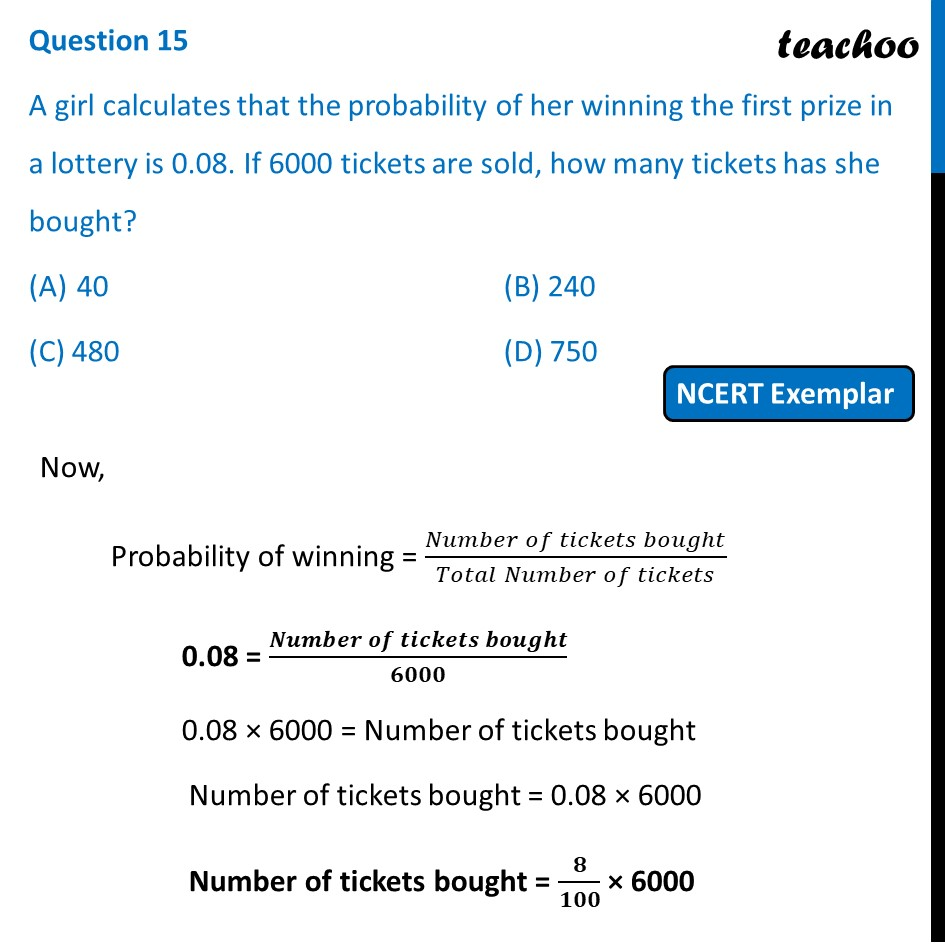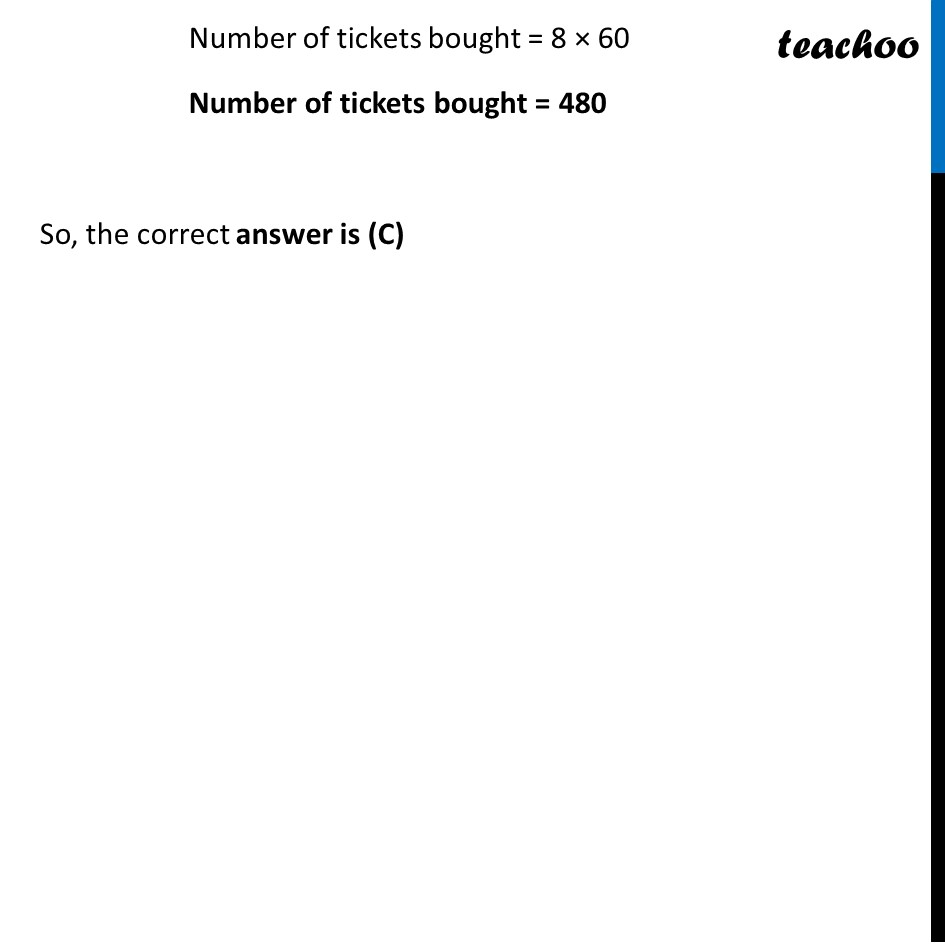## (C) 480          (D) 7501. Chapter 15 Class 10 Probability (Term 1)
2. Serial order wise
3. NCERT Exemplar - MCQ

Transcript

Question 15 A girl calculates that the probability of her winning the first prize in a lottery is 0.08. If 6000 tickets are sold, how many tickets has she bought? 40 (B) 240 (C) 480 (D) 750 Now, Probability of winning = (𝑁𝑢𝑚𝑏𝑒𝑟 𝑜𝑓 𝑡𝑖𝑐𝑘𝑒𝑡𝑠 𝑏𝑜𝑢𝑔ℎ𝑡)/(𝑇𝑜𝑡𝑎𝑙 𝑁𝑢𝑚𝑏𝑒𝑟 𝑜𝑓 𝑡𝑖𝑐𝑘𝑒𝑡𝑠) 0.08 = (𝑵𝒖𝒎𝒃𝒆𝒓 𝒐𝒇 𝒕𝒊𝒄𝒌𝒆𝒕𝒔 𝒃𝒐𝒖𝒈𝒉𝒕)/𝟔𝟎𝟎𝟎 0.08 × 6000 = Number of tickets bought Number of tickets bought = 0.08 × 6000 Number of tickets bought = 𝟖/𝟏𝟎𝟎 × 6000 Number of tickets bought = 8 × 60 Number of tickets bought = 480 So, the correct answer is (C)